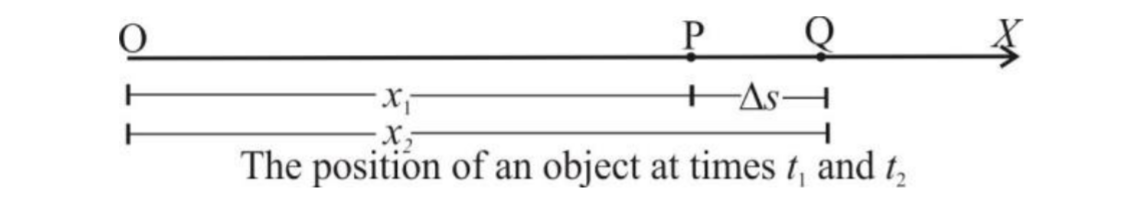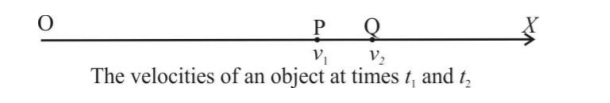# Kinematics - Introduction⁕ Kinematics

↪ Kinematics is a branch of mechanics which deals with the study of motion of an object without reference to mass or forces affecting the motion.

⁕ Distance and Displacement

↪ The distance is different from displacement.↪ The length of the actual path covered between the initial and final positions of an object irrespective of direction is called a distance.
↪ The shortest distance between the initial and final positions in a particular direction is called a displacement.
↪ So the distance is a scalar whereas the displacement is a vector.

↪ Suppose an object has to move from initial position P to final position Q.↪ It may take various paths like path (PRQ), path (PQ) and path (PSTQ).
↪ It is obvious that the paths with length (PR + RQ) and (PS + ST + TQ) are distances and the path with length (PQ) and a specified direction (due east) is a displacement.
↪ The distance divided by time gives the speed whereas the displacement divided by time gives the velocity of the object.

⁕ Average and Instantaneous Velocity
↪ The average velocity of an object is defined as the total distance travelled divided by the time taken in a particular direction.↪ The instantaneous velocity of the object is defined as its velocity at a particular point of its path or at a particular instant of time.
↪ So it is the limiting value of the average velocity when the time interval approaches zero.
That is,⁕ Average and Instantaneous Acceleration
↪ The average acceleration of an object is defined as the rate of change of the average velocity.↪ Suppose an object moving along the x-axis gains velocities ν1 and ν2 at times t1 and t2 respectively.

↪ Thus the average acceleration is↪ The instantaneous acceleration of the object is defined as its acceleration at any instant of time or at any point of its path.
↪ So, it is the limiting value of the average acceleration when the time interval approaches zero.
↪ That is, the instantaneous acceleration is⁕ Displacement-time Graph or s-t Graph

↪ A graph of the displacement of an object and time is called a displacement-time graph or s-t graph.

⁕ Velocity-time Graph or v-t Graph

↪ A graph of the velocity of an object and time is called a velocity-time graph or v-t graph.